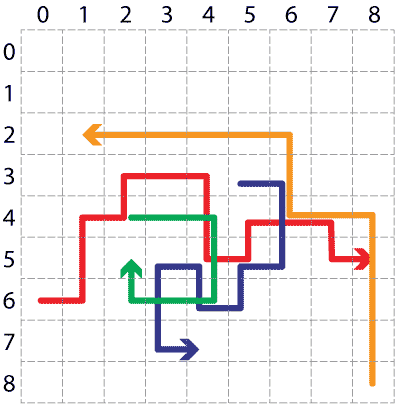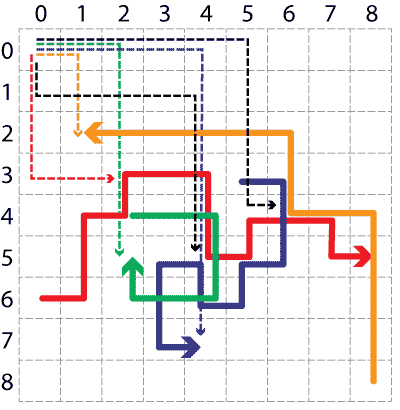## English translationIt turned out that Martians occasionally kidnap people. This homework is about rescuing them.

First the general idea. Although Martian ships are, as we know, circular, the cellar where they keep hostages are rectangular. Every room (except those on the outside) have doors into the neighbouring four rooms. Doors open every 10 second for a moment, hence passing a room always takes 10 seconds. (Hostages are sedated and can't escape despite open doors).

Once an hour, Martian guards teleport into some rooms and walk along some path. These rooms and paths are always the same. We'll get exact data from MOSSAD; the picture shows an example.

We are not sure about the exact rooms where the prisoners are kept, but we assume they are in one of the most visited rooms. There are two such rooms in the picture, (6, 4) in (4, 5); they are visited three times each.

At the exact same moment when guards drop in, Slovenian special forces will teleport into the room (0, 0). Each will walk along some designated path.• If our commando meets a Martian, it grabs him/her/they by the air and keeps him in that room.
• If two commandos enter the room with a Martian at the same time, the one who appears earlier in the list of commandos will stay with the Martian.
• In the end (when all commandos either met a Martian and are keeping him, or the walked till the end of their designated paths), all Martians must be apprehended and there must be a commando in every potential room with hostages. There may be additional commandos present, but this is unimportant.

At the end, we teleport commandos and hostages back home, and also kidnap the Martians, for a good measure.

An example of successfully planned action is on the picture.

The complete simulation is your task for grade 9. For lower grades, you will write function that are partially related to it. Some of them may help you, some or there just so that you can print something out, or draw a situation.

Paths are given like this: "vv^^^^>>v<", where each character represents a move. As you can see from the illustration, the coordinate y increases when going downwrds. Paths of Martian guards from the above images are therefore:

poti_s_slike = [
(0, 6, ">^^>^>>vv>^>>v>"),  # red
(2, 4, ">>vv<<^"),  # green
(5, 3, ">vv<v<^<vv>>"),  # blue
(8, 8, "^^^^<<^^<<<<<"),  # orange
]


• Write function koraki(x, y, pot), which gets the initial coordinates and a path, and returns a list of coordinates visited by someone walking that path.

Call koraki(10, 80, "vv>>>^<") returns [(10, 80), (10, 81), (10, 82), (11, 82), (12, 82), (13, 82), (13, 81), (12, 81)].

• Write a function cilj(x, y, pot), which returns the coordinate of the final room on the given path.

Call cilj(3, 6, "<<v") returns (1, 7)

• Write a function cilji(opisi), that returns a list of triplets (x, y, pot) representing the initial coordinates and paths. The function returns a list of coordinates of final rooms.

Call cilji([(3, 6, "<<v"), (2, 1, "vv"), (5, 5, "")]) returns [(1, 7), (2, 3), (5, 5)].

• Write a function obiskana(x, y, pot) that returns a set of coordinates of visited rooms.

Call obiskana(4, 4, "^^>vv<<") returns {(4, 4), (4, 3), (4, 2), (5, 2), (5, 3), (5, 4), (3, 4)}.

• Write a function najveckrat_obiskana(opisi), which gets the same arguments as cilji and returns a set of most visited rooms. If the same guard visits a room multiple times, this counts as multiple visits.

Call najveckrat_obiskana(poti_s_slike), where poti_s_slike are paths from the illustration, returns {(4, 5), (6, 4)}.

Commandos will be marked with capital letters of the English alphabet (A, B, C, D, E, F, G, H, I, J) and Martians with lower letters. There can be at most ten of each.

• Write a function situacija(specialci, marsovci, sirina, visina), that gets a list of coordinates of commandos, list of coordinates of Martians, and width and height for the map. The function must return a list of list of sets. If the result is named s, then s[y][x] would be a list of people and Martians in the room with coordinates (x, y).

Call

situacija([(1, 0), (0, 2), (3, 1), (0, 2)],
[(2, 2), (3, 1), (3, 1), (1, 1)], 4, 3)


returns

[[set(),       {'A'},  set(),     set()          ],
[set(),       {'d'},  set(),     {'C', 'c', 'b'}],
[{'D', 'B'},  set(),  {'a'},     set()          ]]

• Function znak(m) gets a set of letters.

• If the set is empty, it returns ".".
• If it contains a single element, it returns that element.
• If it contains multiplt elements, it returns a string with the number of elements. You may assume that the size of the set will be at most 9.
• Function izris(polozaj) gets a list of lists of sets, like returned by situacija, and returns a string that would be printed like this:

.A..
.d.3
2.a.


The actual string returned by the function is, of course, ".A..\n.d.3\n2.a.". What we see above would be shown if we print it out.

• Function animacija0(x, y, pot, sirina, visina) gets initial coordinates, path, and width and height. It must return a list of strings that represent consecutive situations at each step. The person walking the path is represented by letter A (as if he were a commando).

Call animacija0(1, 1, "<^>>", 3, 3) returns ['...\n.A.\n...', '...\nA..\n...', 'A..\n...\n...', '.A.\n...\n...', '..A\n...\n...'].

• Function dopolnjeno(s, n) gets list s and returns a new list of length n (where n is at least len(s)). The resulting list contains all elements of s, followed by as many repetitions of the last element of s as necessary to achieve the requested length.

Call dopolnjeno(["Ana", "Berta", "Cilka"], 5) returns ["Ana", "Berta", "Cilka", "Cilka", "Cilka"].

• Function razporedi(specialci, marsovci) gets two lists, one for commandos, one for Martians. The list contains triplets (x, y, pot), describing the initial coordinates and the path taken by a commando or Martian. The function must return two lists of equal length. Their lengths equal the length of the longest path taken by any of the commandos or Martians. Each element of a list contains the coordinates of all commandos or Martians at the given moment.

For illustration, let's see the result of call

razporedi([(0, 2, ">>>"), (3, 3, "<v"), (4, 2, "")],
[(1, 2, "^^<>>"), (1, 1, ">>")])


It must return

([[(0, 2), (3, 3), (4, 2)],
[(1, 2), (2, 3), (4, 2)],
[(2, 2), (2, 4), (4, 2)],
[(3, 2), (2, 4), (4, 2)],
[(3, 2), (2, 4), (4, 2)],
[(3, 2), (2, 4), (4, 2)]],
[[(1, 2), (1, 1)],
[(1, 1), (2, 1)],
[(1, 0), (3, 1)],
[(0, 0), (3, 1)],
[(1, 0), (3, 1)],
[(2, 0), (3, 1)]])


Let us first observe the first list. Its first element contains the initial positions of all commandos, [(0, 2), (3, 3), (4, 2)]. The next element contains positions after the first step, [(1, 2), (2, 3), (4, 2)]: the first command moved right, the second left, and the third one didn't move because his path is emptyh. The third element contains coordinates after the second step, the fourth element the coordinates after the third step... The fifth and the sixth equal the fourth, because commandos make (at most) three steps in this examples.

The second list has the same data for Martians.

• Function animacija(specialci, marsovci, sirina, visina) gets the same arguments as razporedi, and the dimensions of the cellar. It returns a list of strings that are similar to those by animacija0, but for all commandos and Martians.

Call

animacija([(0, 2, ">>>"), (3, 3, "<v"), (4, 2, "")],
[(1, 2, "^^<>>"), (1, 1, ">>")], 5, 5)


returns a list that contains the following strings:

.....
.b...
Aa..C
...B.
.....

.....
.ab..
.A..C
..B..
.....

.a...
...b.
..A.C
.....
..B..

a....
...b.
...AC
.....
..B..

.a...
...b.
...AC
.....
..B..

..a..
...b.
...AC
.....
..B..

• prvo_srecanje(specialec, marsovec) gets two triplets with initial coordinates and paths, representing a path of a commando and a Martian. It returns the coordinate of the first room in which they meet. If they don't meet at all, it returns None.

Call prvo_srecanje((2, 1, ">>"), (1, 1, ">>>>>>")) returns (4, 1): commando first moves in front of the Martian, but then ambushes him in (4, 1).

• bingo(specialci, marsovec) returns similar arguments as the aobve function, except that it contains a list of triplets for commandos. It returns the coordinates of the room where the Martian will first encounter a commando, or None if the little green one will escape.

Call

bingo([(8, 12, ">>>>>>>>"), (10, 14, ">>>>>>>>"), (9, 13, ">>>>>>>>")],
(12, 16, "^>^>^>^>^>"))


returns (13, 14): after three steps, the Martian is at (13, 14), and the commando that starts at (10, 14) and moves right will be there at the exact same moment.

## Ocena 9

Write a function simulacija(specialci, marsovci), which gets a list of paths for commandos (just strings because they will all start at (0, 0)!) and a list of triplets for Martians. The function returns True if the mission's plan is correct and False if not. For conditions, see the introduction.

## Ocena 10

Write a function strategija(marsovci), which returns the optimal strategy. The argument are paths of Martians. Function must return a list of strings.

• Mission must be successful.
• Each Martian must be aprehended as soon as possible. (Designate a commando for each Martian; he should grab him as soon as possible.)
• We must also meet all kidnapped as soon as possible.
• No extra commandos.
• No extra steps for commandos. If commando meets a Martian, the simulation ignores his/her further steps; the strategy must have no such steps.

Tests will use your function simulacija`, therefore it must be written correctly. When testing your strategy, we shall replace your simulation with ours. It is therefore possible that tests on your machine will pass as it you solved the homework for grade 10, while you will in fact get a grade 9. (Although we try to avoid such situations, note that in most other subject you can never know for sure whether your solution is correct and what grade will you receive.)

Last modified: Sunday, 2 January 2022, 12:38 PM